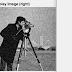Image denoising using method noiseImage denoising is a process of recovering an original image from the noisy image using some denoising method/ filter. Method noise is a technique that processes the unfiltered component of the image.
1. The concept of method noise is used as a post-processing step to enhance the denoising results in terms of quantitative as well as qualitative analysis.
2. The practical implementation of image denoising with and without using method noise is analyzed here.
3. This tutorial explains how the denoising results are effected with and without the use of method noise.
4. The denoising is performed on the "cameraman" image provided by the Matlab itself.
5. The Wiener filtering is used here as the denoising method.
6. The denoising results are tested at the noise variance of 1%.
7. The metrics used to assess the denoising results are MSE, PSNR, and SSIM.
8. The qualitative and quantitative analysis of image denoising with and without method noise are analyzed in the below two sections.
9. The code is written in Matlab using an image processing toolbox.

### Image denoising without using method noise

noisyI = imnoise(I,'gaussian',0,0.01);
figure
imshowpair(I,noisyI,'montage');
title('Original Image (left) and Noisy Image (right)')
denoisedI = wiener2(noisyI,[3 3]);
figure
imshow(denoisedI)
title('Denoised Image')
err = immse(denoisedI,I)
peaksnr = psnr(denoisedI,I)
ssimval = ssim(denoisedI,I)

Fig 1. Denoising results without method noise

### Image denoising using method noise

noisyI = imnoise(I,'gaussian',0,0.01);
figure
imshowpair(I,noisyI,'montage');
title('Original Image (left) and Noisy Image (right)')
denoisedI = wiener2(noisyI,[3 3]);
J = noisyI - denoisedI;
denoisedII = wiener2(J,[3 3]);
denoisedI = denoisedI + denoisedII;
figure
imshow(denoisedI)
title('Denoised Image')
err = immse(denoisedI,I)
peaksnr = psnr(denoisedI,I)
ssimval = ssim(denoisedI,I)

Fig 2. Denoising results with method noise

### Comparative analysis (with and without the use of method noise)

1. The comparative impact of the use of method noise is shown in this section.
2. In terms of qualitative analysis, the denoised image of Fig 2. is far less blurred than the denoised image of Fig 1. So the visual appearance of the denoised image in Fig 2 is better than the denoised image of Fig 1. Hence the denoised results using method noise are qualitatively better.
3. The comparative quantitative analysis is done in the below Table 1. The bold values depict better results. By the use of method noise, MSE value is reduced from 155.4472 to 108.4948, PSNR is increased effectively from 26.2150 to 27.7767, and the SSIM is increased from 0.6236 to 0.7226. So there is a significant increase in the results (metric values) that proves the effectiveness of method noise in the field of image denoising.
4. The concept of method noise is highly efficient and capable of making big good changes in the denoising results only if it is used in a smart and intelligent way.
Table 1. Quantitative analysis of denoising results with and without the use of method noise
 Quantitative analysis Image denoising without method noise Image denoising with method noise MSE 155.4472 108.4948 PSNR 26.2150 27.7767 SSIM 0.6236 0.7226

NOTE: IN CASE OF ANY CONFUSION CONTACT US OR RUN THESE CODES DIRECTLY ON THE MATLAB AND GET THE SAME RESULTS BUT NEVER FORGET TO CITE OUR BELOW RESEARCH PAPERS IN YOUR RESEARCH PAPERS.

1. Prabhishek Singh , Raj Shree, Manoj Diwakar, “A new SAR image despeckling using correlation based fusion and method noise thresholding”, Journal of King Saud University – Computer and Information Sciences (2018), https://doi.org/10.1016/j.jksuci.2018.03.009.
2. Prabhishek Singh , Raj Shree, “A new SAR image despeckling using directional smoothing filter and method noise thresholding”, Engineering  Science  and  Technology,  an International Journal, Elsevier, (2018), https://doi.org/10.1016/j.jestch.2018.05.009.
3. Prabhishek Singh , Raj Shree, “Impact of Method Noise on SAR Image Despeckling”, International Journal of Information Technology and Web Engineering (IJITWE), IGI Clobal, Volume 15, Issue 1, Pages 52-63, 2020.
4. Manoj Diwakar, Prabhishek Singh, "CT image denoising using multivariate model and its method noise thresholding in non-subsampled shearlet domain", 2020, Journal Biomedical Signal Processing and Control, Volume 57, Publisher: Elsevier.

For more image processing topics refer: https://www.gofastresearch.com/
Impact of method noise on image denoisingReviewed by Dr. Prabhishek Singh on April 22, 2020 Rating: 5

1.2.